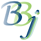# SQL — Numeric Functions

The table below lists the numeric functions specified in the ODBC 3.51 and JDBC 2.0 specifications that are supported by the BASIS DBMS.

Arguments denoted as numeric_exp can be the name of a column (of any numeric type), the result of another scalar function that returns a numeric value, a mathematical operation, or a numeric literal.

Arguments denoted as float_exp can be the name of a column (of type FLOAT, DOUBLE, REAL, or DECIMAL), the result of another scalar function that returns a float value, a mathematical operation, or a numeric literal.

Arguments denoted as integer_exp can be the name of a column (of type signed or unsigned INTEGER), the result of another scalar function that returns an integer value, a mathematical operation, or an integer literal.